# Newton’s 3rd Law of Motion

Newton’s Third Law is very popular law and you must have probably heard some version of it before studying it in your course.

Let us see what the law states.

Statement: All forces come in pairs. If Object 1 exerts a force on object 2 then object 2 must exert a force back on object 1 which is equal in magnitude and opposite in direction.

So if object 1 exerts a force F12 on object 2, then object 2 will exert a force F21 on object 1 which has the same magnitude as F12 but is opposite in direction.

So,

Here

denotes force on object-y by object-x.

Let us see an example: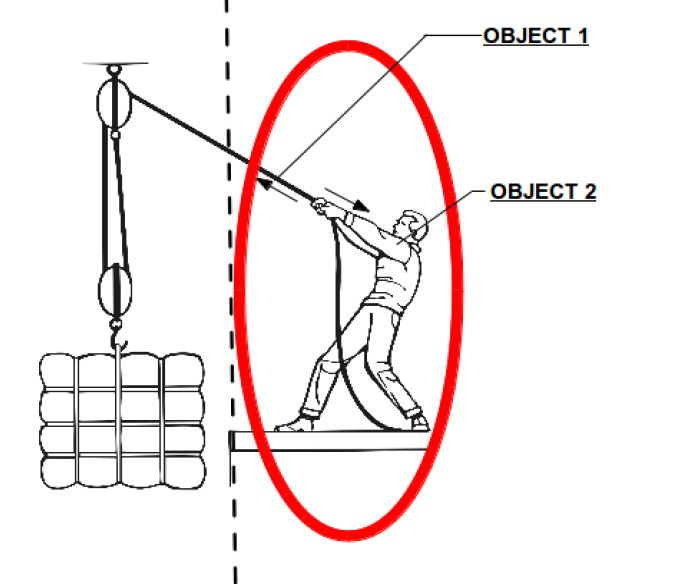If we consider the rope as object-1 and the person as object-2 , we can see that the person is applying a force on the rope (indicated by arrow) and the rope in turn is pulling the person in the opposite direction!

If we consider the same scenario, but this time considers the load and the rope on the left, we will see that the load is pulling the rope downwards but the rope in turn is applying an equal magnitude of force upwards on the load!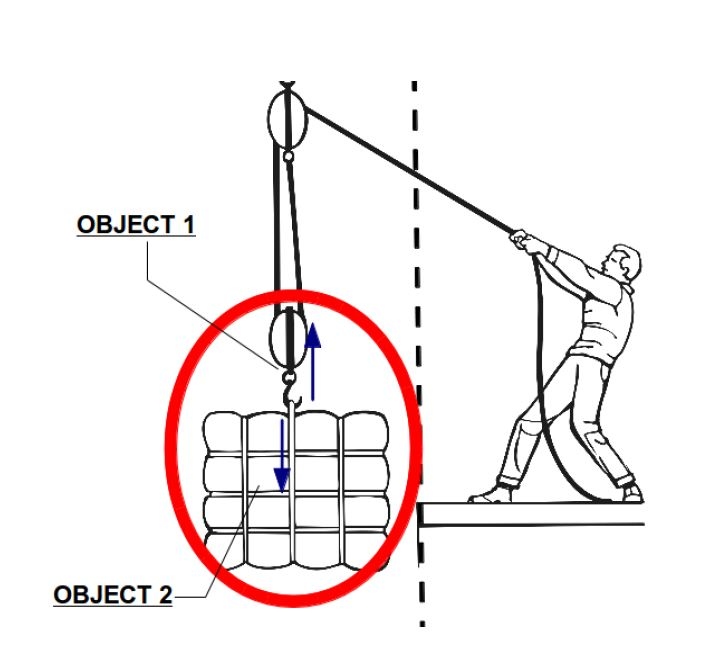We must understand the concept that force is an interaction between two objects!

And in this interaction, the two bodies apply equal force on each other but in opposite direction! Thus force always comes in pair.

Let’s see another example.

You can see a group of people rowing. They are effectively trying to push the water backwards. The water in turn provides a forward force and that pushes the boat forward!

There are lots of other examples where we can clearly see this law at work.

1. When we jump: if you notice carefully next time you jump, you are trying to basically push the ground below your feet before you lift into the air. When we exert force on the ground there is an equal but opposite force from the ground exerted on us! This force that ground exerts on us lifts us into the air!
1. When we swim: when we swim, we use our hands to push back the water and thus we use the reaction force from the water to move forward.

## Action-Reaction Pairs

As we noted force always occurs in pairs.

So we often call them action-reaction pairs.

They are always equal in magnitude! But they act on different objects. If the force on object-2 by object-1 is called action, then the force exerted back by object-2 on object-1 will be called the reaction force.

For example if you try and kick a small stone with your foot (consider this a thought experiment!!) the stone will move away due to the force exerted on it by your foot. But you will also end up hurting your foot due to the reaction force by the stone.

Sometimes the mass difference in the two bodies or objects is so huge, that out of the pair of forces only the effect of one is noticeable!

For example the earth exerts a force of gravity on us. According to Newton’s third law then we must exert a force on earth also! In fact we do!!

But the earth’s mass is so huge, that the resultant acceleration on earth (a=F/m), is negligibly small.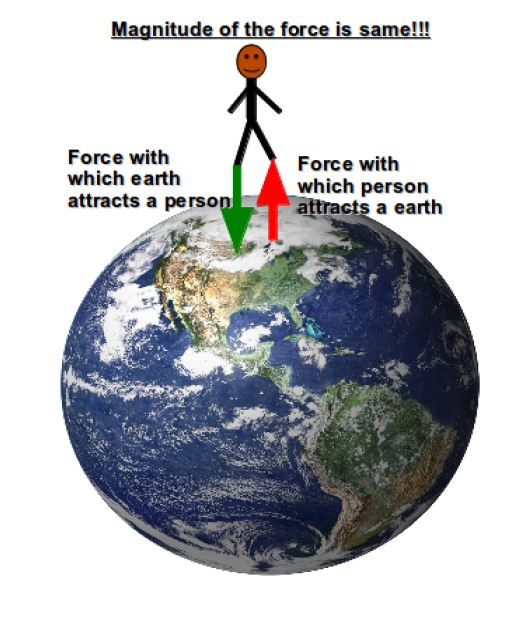So in every scenario, the Third law is always valid. For every force there is always a reactionary force equal in magnitude and opposite in direction.

## Why Don’t action-reaction forces cancel each other?

This confusion often arises when the Third law of motion is discussed.

But for the Net force to be zero (or the forces to cancel each other out), the pair of forces (action-reaction pair) must act on the same object. But they DO NOT act on the same object! So they DO NOT CANCEL out each other!!

Let’s see an example of the gravitational interaction of the sun and earth.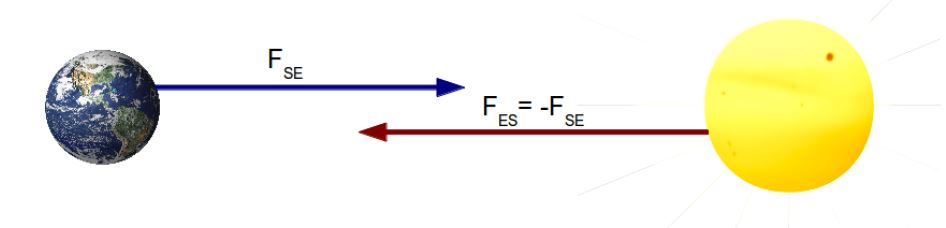Now if we draw the FBD of the earth, we get: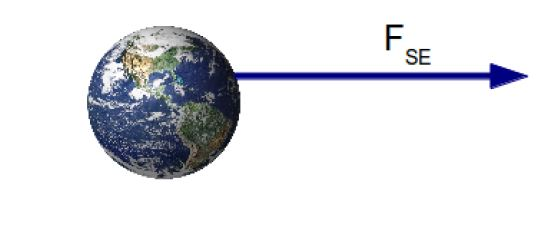Thus we see that the Net Force is not zero.

A FBD for the Sun would display a similar scenario.

Thus we see that the forces (Action-Reaction) act on different bodies. But their magnitudes and direction are related by the Third Law!

[box type=”success” align=”” class=”” width=””]You can see Prof. Dan Fullerton’s lecture on Newton’s 3rd Law of Motion, where he discusses and explains the Third law of Motion.[/box]

SPECIAL OFFER!

SUCCESS8